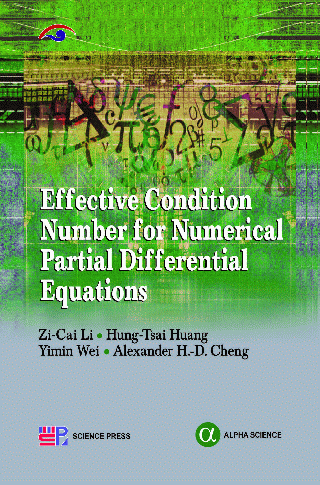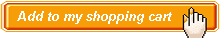Effective Condition Number for Numerical Partial Differential Equations Authors:   Zi-Cai Li, Hung-Tsai Huang, Yimin Wei, Alexander H.-D Cheng ISBN: 978-1-84265-913-7 Publication Year:   2014 Pages:   286 Binding:   Hard Back About the book For a numerical method, its stability is a crucial issue in the sense that an unstable algorithm can render it useless in practical computation. For an over-determined linear algebraic equation , its stability is typically evaluated by using the traditional condition number, Cond= , where and are the maximal and the minimal singular values of matrix , respectively. In this book, the concept of an effective condition number, Cond_eff= , is introduced. Cond_eff is smaller, and generally much smaller, than Cond, and is a better stability criterion. The Cond_eff can be used as an estimation on stability for the numerical solutions of partial differential equations (PDEs) using algorithms, such as the collocation Trefftz method, the spectral method, the finite difference method, and the finite element method. An analysis of stability integrated with errors is the focus of this book. Key Features Table of content Preface / Acknowledgments / Effective Condition Number / Collocation Trefftz Methods / Simplified Hybrid Trefftz Methods / Penalty Trefftz Method Coupled with FEM / Trefftz Methods for Biharmonic Equations with Crack Singularities / Finite Difference Method / Boundary Penalty Techniques of FDM / Boundary Singularly Problems by FDM / Finite Element Method Using Local Mesh Refinements / Hermite FEM for Biharmonic Equations / Truncated SVD and Tikhonov Regularization / Appendix: Definitions and Formulas / Epilogue / Bibliography / Index.Audience Undergraduate and Postgraduate Students, Teachers and Professionals